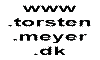> Paradoxes and paradigms > c + c = c ?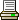Paradoxes and paradigmsParadoxes in PhysicsSe også:Udskriv siden
 Paradoxes and paradigms in Physics c + c = c ?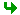The Galilei TransformationInertial systems and the principle of relativityThe paradox of light and the 'ether'No to the 'ether', yes to the principle of relativityEinstein's assumptionsTime intervals are relativeLengths are relativeThe Muon ParadoxThe Twin ParadoxThe Lorentz TransformationThe correspondence with Classical Physics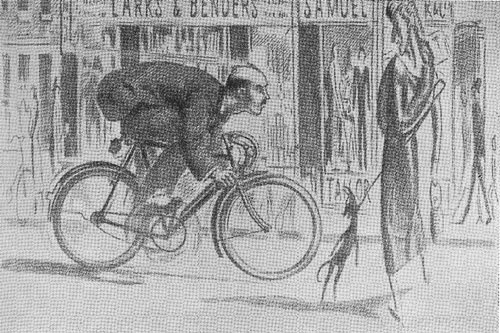Illustration from: "Mr. Tompkins in Wonderland or stories of c, G and h", George GamowThe Galilei transformation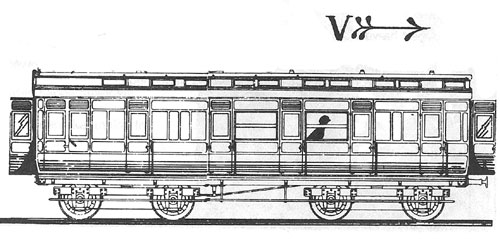Drawing from Joseph Schwartz & Michael McGuinnes: "Einstein for beginners"

A train runs with the speed v = 100  km/h.
A passenger inside the coach walks in the same direction as the train with speed w = 3 km/t in relation to the coach.
Then the passenger moves with speed u = w + v = 103 km/h in relation to the ground.
If the passenger walkd in the opposite direction of the direction of the train then the passenger moves with speed u = 97 km/h in relation to the ground.
This principle - to add velocities (with sign) - is named the Galilei-transformation (Galileo Galilei 1564-1642).The law of inertia, inertial-systems and the principle of relativity

Galilei and later Isaac Newton (1642-1727) formulated the law of inertia:
An object not influenced by external forces will continue in a state of rest or uniform rectilinear motion.

This law can also be turned in a definition of an 'inertial-system':
An inertial-system is a system in which the law of inertia is valid.

But is the law of intertia always valid?
No, not for instance in a train accelerating or turning in a  bend, or in a merry-go-round, or on the Earth spinning around its own axis.(Anyway, the law of inertia can be "saved" by introducing so-called 'fictitious forces').

When recognizing one inertial system you have infinitely many of them. Each other system moving with uniform rectilinear motion in relation to the original inertial system must itself be an inertial system.

In inertial systems the principle of relativity is valid (originally formulated by Galilei og Newton):
All laws of Physics are having the same form in all inertial systems.
Or:
It is not possible internally within an inertial system to decide if this system is at rest or moving with uniform rectilinear motion.
Only by 'looking out' is it possible to decide if your 'own' inertial system is moving in relation to another inertial system.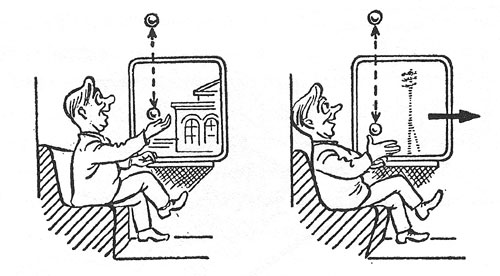Drawing from L.D.Landau & G.B.Rumer: "What is Relativity?"
Several of the examples on this webpage are inspired by this book.

In a train in uniform rectilinear motion it is not possible for a passenger by doing experiments in the coach - like throwing a ball vertically up - to decide if the train is moving or at rest. Only by looking out the window can it be decided if the train moves in relation to the ground/platform or not. The train is an inertial system.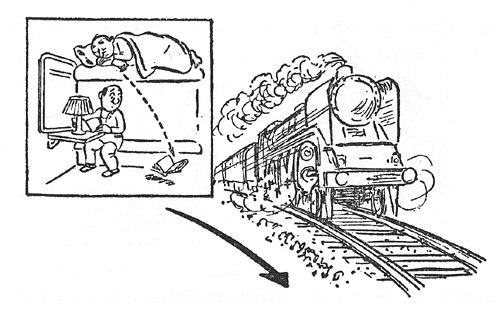Drawing from L.D.Landau & G.B.Rumer: "What is Relativity?"

It is a totally different situation if the train is moving in a curve. That can be decided without looking out the window, like on the drawing. Anytime the train is having an acceleration, that is changing its velocity (by changing speed or direction, e.g. by moving in a curve) the train is no more an inertial system.

We must note that the principle of relativity is a well founded principle of classical Physics.The paradox of light and 'the Ether'

James Clark Maxwell (1831-1879) formulated 'Maxwell's equations of electromagnetism'. One of the solutions of his equations was especially interesting:
Oscillating electric and magnetic fields create an 'electro-magnetic wave', and the speed of that wave is 300.000 km/s. Light is an example of such an electro-magnetic wave.

Another consequence of Maxwell's equations is that the speed of light do not depend on the speed of the emitter of the light - in contrast to the speed of a rifle bullet which must depend on the speed of the weapon (like addition of velocities in the former passenger-train example).

Also sound has this property, that the speed of sound do not depend on the speed of the source of the sound. The explantion of this is that sound propagates through a medium, e.g. air, and that the speed of sound is fixed in relation to this specific medium.

It is therefore tempting to imagine that light - just like sound - must propagate through an until now unknown 'medium' which then must permeate everything in the universe (we can see the stars!). This medium was named 'the Ether'.

But if that is so, then the principle of relativity cannot be valid! Because then it becomes possible inside the coach to decide if the train moves or not by doing an experiment with light:

 In a train at rest the speed of light is 300.000 km/s in relation to both train and platform. If this train now is moving (through the Ether) with the speed 240.000 km/s, then a ray of light emitted from a lamp in the train in the same direction as the train is moving will have the speed 300.000 km/s in relation to the platform and the Ether, but will have the speed 300.000 - 240.000 = 60.000 km/s in relation to the train. Similarly a ray of light emitted in a direction opposite to the direction of the train will have  the speed 300.000 + 240.000 = 540.000 km/s in relation to the train. So by measuring the speed of light emitted form a source in the train it can be decided if the train moves (and in which direction it moves).

(Notice that this experiment could not be done by fireing a rifel bullet from a weapon in the train, because the speed of the bullet depends on the speed of the emitter of the bullet).

Objection: Why not imagine that the Ether is simply following the movement of the train?Then the speed of light would be 300.000 km/s in relation to the train in all direction, no matter if the train moves or not. And then the principle of relativity would be saved.
- Well, actually this would be inconsistent with experiments done which confirm that light do not take part in the movement of the medium in which it is propagating, that medium for instance being moving water.

The idea of the Ether is of course rather strange. That kind of theory is sometimes by physicists and philosophers named an 'ad-hoc theory' - that is a theory which 'on occasion' is suggested with the purpose of solving a very specific problem, but at the same time a theory wich is not connected to the larger more coherent system of theories relevant to this cathegory of problems, and a theory not leading to new knowledge. The cost of the Ether-theory is giving up the principle of relativity. So it is more tempting to give up the idea of the Ether, but how then explain the speed of light in relation to different inertial systems?Rejecting the Ether-theory experimentally and saving the principle of relativity

In 1883 Michelson and Morley investigated experimentally if the speed of light as measured in a laboratory depends on the ray being perpendicular or parallel to the direction of the Earth's movement around the Sun. If the Ether exists then an effect of the 'Ether-wind' would be that there should be a difference between these two measured velocities. The experiment showed no difference.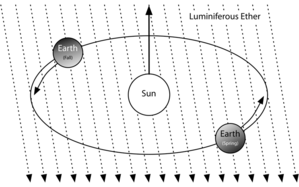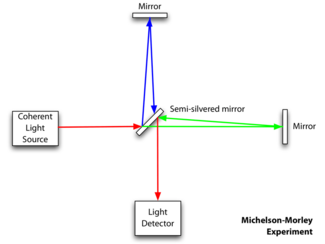Drawings from: http://en.wikipedia.org/wiki/Michelson_Morley_experiment (29-11-2005)

Regarded as an attempt to prove the existence of the 'Ether-wind' this experiment failed. But this 'zero-result' instead supported the assumption that the propagation of light is not in conflict with the principle of relativity. On the other hand, we then have no understanding of the propagation of light - we are not able to apply the Galilei transformation and add or subtract the speed of light as we did in the former train-example. The speed of light seems to be an absolute entity in contrast to all other velocities. The reason for this apparent paradox could be that we are asuming some conditions ('paradigms') which are not correct. Maybe we are, without knowing it, assuming something else to be absolute which really should be treated in some relative way? What can this 'something' be? We have a suspicion. Velocities are calculated as distance travelled over time-intervals, so if velocities are queer, then also distance and time could be queer - og maybe in some way relative?

By the way, this experiment of Michelson and Morley is interesting from a history-of-science point of view. If this experiment had been within reach at the time of Galilei, then the catholic church could have referred to the result as a verification of the theory, that the Earth stands still, and as a falsification of the theroy of Galilei, that the Earth circles the Sun. In the evolution of science experiments and theory must folow each other in a continuous interaction.Einsteins assumptions

In 1905 Albert Einstein (1879 - 1955) published an article in the German Physics-journal 'Annalen der Physik'. The title of the article was: 'Zur Elektrodynamik bewegter Körper' ('On the electrodynamics of moving bodies').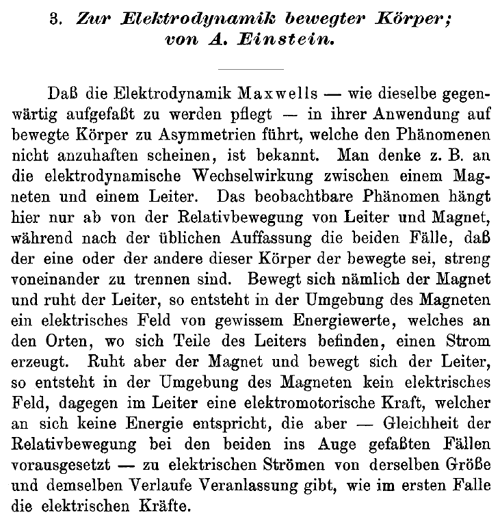On the Electrodynamics of moving bodies

Einstein demonstrates that Maxwell's electromagnetic equations do not keep the same form when transformed via the Galilei-transformation between different initial-systems. This is in conflict with the principle of relativity. If we again do not want to give up the principle of relativity, then:

Either something is wrong with Maxwell's theory or something is wrong with the Galilei-transformation.

Einstein's choice was to try to solve this problem by making two assumptions (in the same time rejecting the Ether-theory - or rather making it superfluous):

1. The speed of light in vacuum is c = 300.000 km/s and independent of both the speed of the emitter and the receiver.
2. The principle of relativity is valid in all inertial systems.

The consequence of these two assumptions will be that we must give up other 'old' assumptions:

1. Time and space are not absolute, but relative entities.
2. The Galilei-transformation must be substituted by another kind of transformation.

Maxwell's theory survives, but Newton's classical theory of kinematics and mechanics must be revised, because that theory has the Galilei-transformation in its foundation. However, we must expect that the well-confirmed Newtonian Physics still can be applied in the 'classical borderland' of velocities small in relation to the speed of light.Time-intervals are relative

 We are passengers in a special Einstein-train travelling with a speed of 240.000 km/s = 0,24 mill.km/s. The train leaves railway station 1 and travels 864 mill.km to railway station 2. The trip lasts 1 hour (864/0,24 = 3600 s). When we enter the train at railway station 1 we synchronize our watch with the station-clock. Arriving at railway station 2 we realize that our watch is almost an half hour slow in relation to the clock at railway station 2.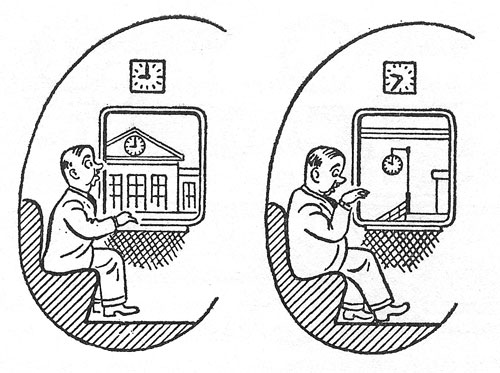Drawing and number-example from L.D.Landau & G.B.Rumer: "What is Relativity?".
The idea of the 'light-clock' is probably from R. P. Feynman

 Inside our coach of the train we (the passenger) do an experiment. We transmit a ray of light up to the ceiling from a lamp fixed to the floor of the coach. In the ceiling we fix a mirror to reflect the ray back to the lamp. The ray's departure from and return to the lamp are two 'space-time-events' which are well defined both as seen from us (the passenger) and from a person standing on the platform.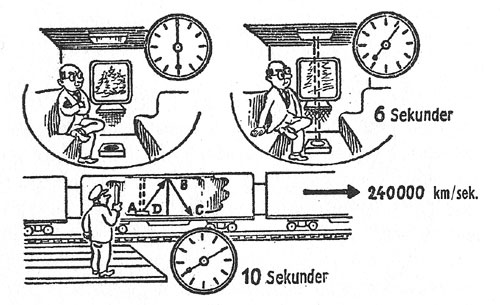Drawing from L.D.Landau & G.B.Rumer: "What is Relativity?".
An error in the triangle is corrected here in relation to the original drawing.

 From the our (the passengers) point of view the ray of light moves verically up and vertically down, the route being D-B-D. Do the geometry of the ray as seen inside the coach depend on the train moving or not? No, according to Einstein's assumption 2, the principle of relativity. From the point of view of a person on the platform the ray of light moves along two sides of a isosceles triangle A-B-C. The speed of light is c both from our (the passengers) and from the platform-person's point of view. According to Einstein's assumption 1. The ray of light moves a longer distance as seen from the platform than as seen from the train, but with same speed. Therefore the time-interval between the two space-time-events as seen from the platform (t) must be longer than the time-interval as seen from the train (t').

 We now calculate the time-intervals between the two space-time-events as seen from respectively from us (passenger in train) and from the person at the platform. The speed of light is 0,3 mill.km/s The speed of the train is 0,24 mill.km/s From the passenger's point of view the ray of light travels from floor to ceiling and back from ceiling to floor, that is the route D-B-D. The time-interval for this movement is t'. Then(*): t' = 2BD/0,3 eller BD = ½ x 0,3 x t'. From the platform-person's point of view the lamp on the floor of the coach trravels the route A-D-C during the same time-interval used by the ray of light travelling the route A-B-C. The time-interval for this movement is t. Then (*): t = (AB+BC)/0,3 = AC/0,24 Triangle ABC is isosceles, so: t = 2AB/0,3 = 2AD/0,24 or: AB = ½ x 0,3 x t   og   AD = ½ x 0,24 x t Triangle CBD is right-angled, so Phytagoras: AB2 = AD2 + BD2 Joining these equation together we get: (½ x 0,3 x t)2 = (½ x 0,24 x t)2 + (½ x 0,3 x t')2 or: (0,32 - 0,242) t2 = 0,32 t'2 or: (t/t')2 = 0,32 / (0,32 - 0,242) or: t/t' = 10/6 Let us assume that the Einstein-train is BD = 0,9 mill.km in height ! Then: The time-interval as seen from the train: t' = 2BD/0,3 = 6 sek. The time-interval as seen from the platform: t = 10/6 t = 10 sek.

A little more general calculation: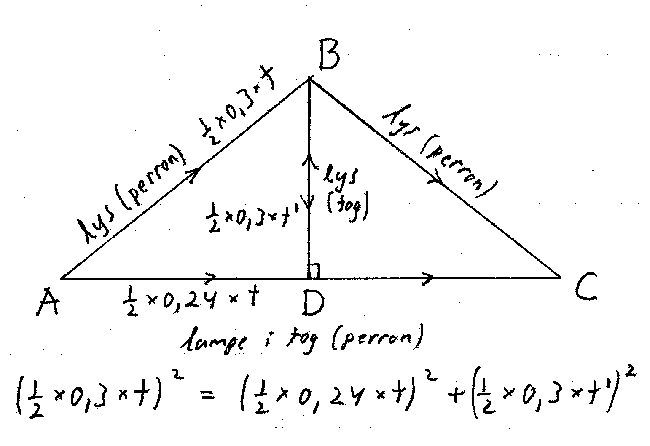The equation for the time-intervals can be changed to: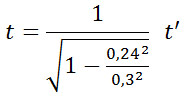In general the result is: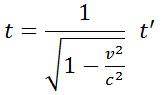Here v is the speed of the train and c the speed of light. The time-interval t' is the time for a process at rest in relation to the observer (the 'rest-time', 'proper time' or 'eigen-time') The time-interval t is the time for the same process having the speed v in relation to the observer. This penomena is named the 'time-dilation' because t > t'.

If the train according to the station-clocks uses 1 hour to travel from station 1 to station 2, then this same travel as seen from our (the passenger's) point of view only lasts 60 min x 6/10 = 36 min. Our watch becomes 24 min slow in relation to the station-clocks.i forhold til stationsurene during this hour.

If the lamp on the coach's floor again reflects the light ray up, etc., then this light-experiment can be interpreted as a clock which is 'ticking' each time the light returns to the lamp. All time-processes must follow this time-transformation. If not, there would be a contradiction between the time-intervals of the experiment and the time-intervals measured by other types of clocks. Moving clocks become slow in relation to clocks standing still. Or to be more precise::

Clocks (processes) moving in relation to an observer will - as seen from this observer - become slow in relation to clocks (same processes) which are at rest in relation to an observer.

The passenger in the train observes a process (ticks of the 'light-clock') to last 6 s., while a person on the platform observes the same process to last 10 s. Therefore the passenger's watch must be slow, and this can be verified by the passenger by comparing the time-interval on his watch with the time-interval between the clock he passes at station 1 and the clock he later on passes on station 2.

But then, is this result not in contradiction with the principle of relativity? Could we not say that the slow clocks are performing a 'more absolute' movement that the fast clocks?
And do we not get a contradiction if we switch over and - in accordance with the principle of relativity - interprete the situation as the passenger in the train observing the person on the platform passing with high speed in the opposite direction?

No, because the two ways of interpreting the situation are not 'symmetric'. We apply not two, but actually three clocks to compare the time-intervals. The passenger compares his watch with two different clocks positioned at two different places (the railway stations 1 and 2). If we really should make the switch correct it would look like this:

The passenger on the train observes the platform passing (in the opposite direction). The person on the platform first passes and observes a clock in the front end of the train, later on a clock in the rear end of the train.

The person on the platform creates a light-watch with 6 s between the ticks. The passenger on the train observes these ticks to last 10 s. The platform-person's clock must therefore be slow, and he can verify this by comparing his watch with the time-interval between the train-clock he passes in the front end of the train with the train-clock he later on passes in the rear end of the train.The lengths are relative

Let us look at the distance our train traveling.

 The distance between Station 1 and Station 2 is 864 mill.km and the trip takes 1 hour when the train's speed is 0.24 mill.km / s But the passenger on the train observes that it only takes 36 minutes or 2160 seconds to see the stretch between the stations passing behind. He must then conclude that the distance between the stations is: 0,24 mill.km/s x 2160 s = 518,4 mill.km So the stretch between stations looks shorter as seen from the train than as seen from the ground. The lenght-shortening factor here is 6 / 10.

 The formula for the lengths are generally as follows: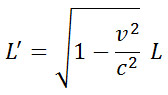where v is the train's speed and c is the speed of light. The length L is the length of a stretch at rest relative to the observer (' rest-length ') The length L' is the length of the same stretch on the move with the speed v relative to the observer. This is called 'length contraction' because L' < L.

The length of an object seems to be shorter when the object moves in relation to an observer compared with the object at rest.

Just like the time dilation this length contraction do not contradict the relativity principle. A person on the platform will similarly observe that the length of a train passing will look shorter than the length of the train as measured by a passenger on the train.

Let us say that the length of the train is 5.4 mill.km, (rest length as seen from the train). As seen from the platform, its length will then be 5.4 mill.km x 6 / 10 = 3.24 mill.km.

But how can the person on the platform, measure this length of the train?

The person on the platform sets up a photoelectric cell which gives an electrical impulse when the train's front end passes and corresponding when the train's rear end passes. This process (from one pulse to another) is at rest relative to the platform, and the time it takes is found to be 13.5 seconds.:
Then the person on the platform concludes the train's length to be:
0.24 mill.km / sec x 13.5 seconds = 3.24 mill.km.

How do we know that the time-interval between the photoelectric impules will be 13.5 seconds?
Let us name this time '?'.

As seen from the train the photocell-process moves backward with the speed of the train, and the time for this process is time-dilated by the factor 10 / 6. So the passenger on the train observes that the time between the the photocell passing first the train's front end and later the trains rear end becomes  ? s x 10 / 6.
But the passenger can also calculate this time-interval by dividing the train's rest length with the speed of the train:
5.4 mill.km / 0.24 mill.km/s = 22.5 s. So now we know that
? s x 10 / 6 = 22.5 sec, or that ? s = 6 / 10 x 22.5 s = 13.5 s.Muons are elementary particles (sometimes called 'heavy electrons') formed when cosmic radiation enters the atmosphere at an altitude of approximately. 10 km above the Earth's surface.

We know from experiments in laboratories that the muon has a lifetime of 2.2 10-6 s (after which the particle decays into other particles).

Muons formed by cosmic radiation can be detected at the Earth's surface. This is a paradox, for the muon do not live long enough to reach down to us - even at the speed of light!

How can this paradox is dissolved? - With the help of relativity theory.

 Muon's lifetime: t' = 2.2 10-6 s, as measured for muons at rest Muon's velocity: v = 0.999 c, where c is the speed of light As seen from a Classical Physics point of view the muon in its lifetime can travel the distance:       L = 0.999 c x 2.2 10-6 s = 0.659 km But 659 meters! - That is not far enough to reach down to us from the height of 10 km where the muons are created. As seen from the muon's rest system the muon's proper lifetime is t'. As seen form the Earth system, this time becomes dilated to t:The factor in front of t' is called 'gamma'. The value of gamma here is: 22.4 We then get the muon's lifetime as seen from the Earth system to be:       t = 22.4 x 2.2 10-6 s = 49.2 10-6 s As seen from the Earth system the muon in this time-interval travels the distance:       L = 0.999 c x 49.2 10-6 sek = 14.7 km - That is far enough to reach down to us. We can also see this from muon's 'point of view': The muon's proper life time is t' = 2.2 10-6 s, and on its journey down towards the Earth the muon 'sees' the stretch of space travel backward with the speed 0.999 c. The length of this stretch, L', is contracted in relation to the same stretch as seen from the Earth, L, and we know that L = 10 km:From this we get the length of the stretch as seen from the muon to be:       L' = 1/22.4 x 10 km = 0.447 km = 447 m. As the muon in its proper lifetime is able to travel the distance 659 m (or rather the stretch travels backward this distance in the muon's proper lifetime), the muon has time to reach down to us.Two twins, Andy and Beatrice, both 18 years old, say goodby to each other on the departure area for spaceships. Twin Beatrice enters the spaceship and travels to a distant star with great speed. Beatrice returns to Earth and Andy welcomes her back. Beatrice finds that she has been traveling in 16 years (her proper time interval t') and reached the age of 34, but her brother has become 20 years older (time interval t) and reached the age of 38! This is confirmed when the two twins compare their watches, Beatrice's watch shows "year 34", Andy's shows "year 38" so Beatrice's watch is 4 years behind Andy's !  If Andy had been able to follow Beatrice's watch, for example during a part of the outward journey, he would also expect this according to the theory of relativity. Beatrice has not observed anything special, she just judge to have been gone for 16 years.

But according to the relativity principle, we could also see the situation from the standpoint of Beatrice. Beatrice sees the Earth and Andy move backward at high speed, and later the Earth and Andy returns towards her. Beatrice must therefore believe that it is Andy who has moved while she was at rest. Therefore it must be Andy who becomes 16 years older while Beatrice becomes 20 years older.

This problem is alike the earlier mentioned problem with the railway travel between respectively the two stations - or the station's travel in the opposite direction. But unlike the previous example involving 3 clocks (a clock moving between two fixed clocks) in twin paradox we have only 2 clocks - the two twins' watches.

Here is apparently a genuine paradox, for the two twins may well in both versions of the story directly compare their clocks at the same spacepoint (where they meet) - and then both results cannot be true. Do Beatrice's watch show "year 24" and Andy's "year 38" or vice versa - or is the problem symmetric so both watches show the same - or something else?

The paradox dissolves when we recognize that we have not kept us within the framework of the theory of relativity - or rather, the special theory of relativity, formulated by Einstein in 1905. This theory deals only with inertial systems, i.e. systems which are moving at uniform rectilinear velocity in relation to each other. To return to Earth Beatrice must in a specific moment turn around and move in the opposite direction. This will alter her direction of movement (she experience an acceleration), and during this, her system is no longer an inertial system.

In the general theory of relativity by 1915 this problem can be understood. According to this theory time and space also change because of gravity fields and accelerations. Taking into account Beatrice's 'turning around' only the first version of the story can be confirmed. Upon arrival Beatrice has become 16 years older and Andy has become 20 years older.

See more: The Twin Paradox revisited

Time-effects of the theories of relativity are often used in science fiction novels (with great skill, for example, by Ursula K. Le Guin). Strange things happen, but if you keep within the range of the theories of relativity, it is not possible to travel back in time and kill your own parents! Fortunately. See the following Limerick poem quoted in Martin Gardner's book on relativity (see literature) as an example of something which can not be done:

There was a young lady named Bright
who traveled much faster than light.
She started one day
in the relative way,
and returned on the previous night.c + c = c ! - and the Lorentz-transformationen

One consequence of the special theory of relativity is that the Galilei transformation must be abandoned and replaced by a new kind of transformation known as the Lorentz transformation. H.A.Lorentz (1852-1928) suggested this new transformation in order to explain that bodies seem to be contracted when they are moving through the ether. Einstein modified Lorentz's equations and interpreted them in a much more general way. These equations do not express an effect of the ether - for the ether does not exist - but they express a characteristic property of space and time.

The previous formulas for time dilation and length contraction are special cases of the Lorentz transformation. The full formula contains a strange blend of space and time not known from the classical physics.

Maxwell's equations prove to be invariant under the Lorentz transformation, ie. they retain their form in all inertial systems. - they did not under the Galilei-transformation. That was one of the main reasons for Einstein to formulate his special theory of relativity. This garanties the principle of relativiy to hold also in electromagnetics.

New transformation formulas for space and time must also imply new formulas for how velocities "add together".

According Galilei-transformation:

u = w + v

where w was the passenger's velocity in relation to the train coach, v the trains velocity relative to the ground and u the passenger's velocity relative to the ground.

According to the Lorentz transformation the "addition" should be done as follows: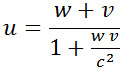Let's take w = ½c and v = ½c. Then: u = c/1.25 = 0,8 c.

Let's take w = c og v = c. Then: u = 2c/2 = c !

The last result is in accordance with Einstein's assumption 1.Correspondence with Classical Physics

What about the Galilei-transformation and the classical physics? Should everything just be thrown away? No, the classic Newtonian mechanics is still OK as long as we stick to speeds which are small relative to the speed of light.

If w and v are small in relation to c, so that the product w v is small in relation to c2 (w v << c2), then w v/c2 tends to 0, and the Lorentz transformation approximates to the Galilei transformation with the good old formula for addition of velocities.

In the general theory of relativity there is similar 'un-classical' effects because of large gravitation fields or high accelerations. But Newton's gravity theory is still working fine if the gravitationsforces are not too strong.

The same applies to the other great theory of modern physics, the quantum theory. For particles (and energy) with large values, the quantum physical results tends towards the results of the classical mechanics. Niels Bohr formulated this in his 'principle of correspondence'.

You could say that modern physics is a revolution in relation to the classical physics, because it replaces the old paradigms, for example concerning space, time, particle orbits and cause-and-effect with new paradigms. But for small speeds and large masses and energies the new physics get in correspondance with the old physics.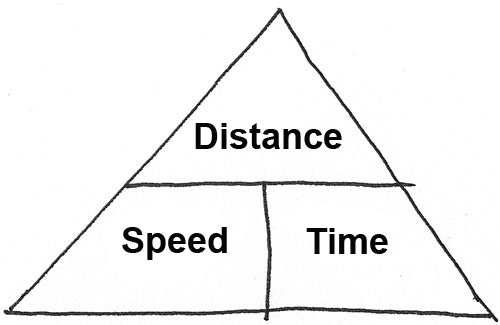(*) "Speed Triangle" speed = distance / time distance = speed x time time = distance / speedLiterature:

L.D.Landau & G.B.Rumer: "What is Relativitet?", Munksgaard, 1963

Martin Gardner: "Relativity for the Million"

George Gamow: "Mr. Tompkins in Wonderland or Stories of c, G and h"

Joseph Schwartz & Michael McGuinnes: "Einstein for Beginners"

Leo Sartori: "Understanding Relativity: A Simplified Approach to Einstein's Theories", University of California Press 1996Opdateret 18-11-2014 , TMSidens top